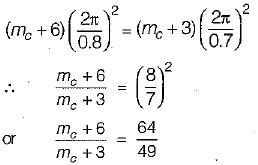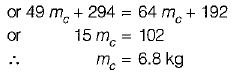# Linear Vibration Analysis - 1

## 10 Questions MCQ Test Topicwise Question Bank for GATE Mechanical Engineering | Linear Vibration Analysis - 1

Description
This mock test of Linear Vibration Analysis - 1 for Mechanical Engineering helps you for every Mechanical Engineering entrance exam. This contains 10 Multiple Choice Questions for Mechanical Engineering Linear Vibration Analysis - 1 (mcq) to study with solutions a complete question bank. The solved questions answers in this Linear Vibration Analysis - 1 quiz give you a good mix of easy questions and tough questions. Mechanical Engineering students definitely take this Linear Vibration Analysis - 1 exercise for a better result in the exam. You can find other Linear Vibration Analysis - 1 extra questions, long questions & short questions for Mechanical Engineering on EduRev as well by searching above.
QUESTION: 1

Solution:
QUESTION: 2

### Select the WRONG statement:

Solution:

In free or natural vibrations, no force acts on the body after an initial displacement has been imparted to it.

QUESTION: 3

### What is the maximum acceleration of a particle which moves in simple harmonic motion with an amplitude of 3 mm and frequency of 20 Hz?

Solution: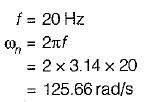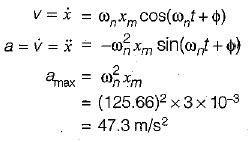QUESTION: 4

The equation of free vibration in a system is

x + 49π2x = 0

Its natural frequency is

Solution: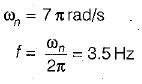QUESTION: 5

A 32 kg block is attached to a spring and can move without friction in a slot as shown.The block is in its equilibrium position when it is struck by a hammer, which imparts to the block an initial velocity of 250 mm/s.What is the amplitude of motion?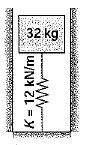Solution: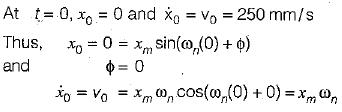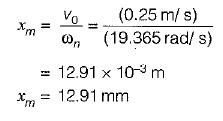QUESTION: 6

A 12 kg block is supported by the spring shown. If the block is moved horizontally rightward from its equilibrium position and released with its amplitude of 50 mm, what is the maximum velocity of block?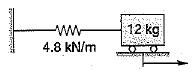Solution: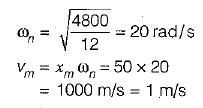QUESTION: 7

A 5 kg block, attached to the lower end of a spring whose upper end is fixed, vibrates with a period of 6.8 s. What is the period'of vib ration of a 3 kg block which is attached-to the center of the same spring if the upper and lower ends of the spring are fixed?

Solution: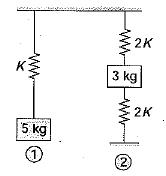Spring constant is inversely proportional to its length

K1 = K
K2 = 2K + 2K = 4K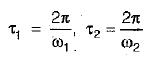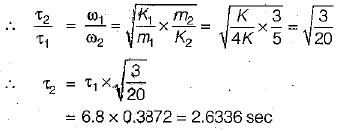QUESTION: 8

When there is a reduction in amplitude over every cycle of vibration, then the body is said to have

Solution:

When no external force acts on the body, after giving it an initial displacement, then the body is said to be under free or natural vibrations. The frequency of the free vibrations is called free or natural frequency.
When there is a reduction in amplitude over every cycle of vibration, the motion is said to be damped vibration.

QUESTION: 9

Block ‘A ’ of mass m is s upported by the spring arrangement as shown. If the mass of pulley is negligible and block is moved vertically downward from its equilibrium position and released, what is the frequency of motion?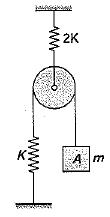Solution: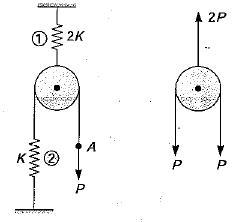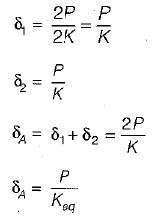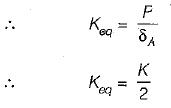and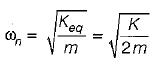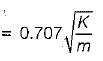QUESTION: 10

The period of vibration of the system shown is observed to be 0.8s. If block lA' is removed, the period is observed to be 0.7s. What is the mass of block ‘C’?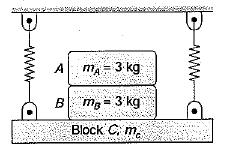Solution: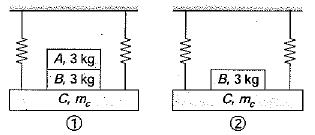we have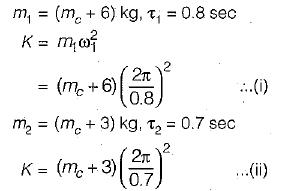From equation (i) and (ii),